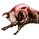3791 views
The usage is very easy. When the line is green you can open long position, when the line is red you can open short position and when it's black just check by yourself.
Usually I use it with RSI and Bollinger Bands, in order to determine when the signal is strong or weak.

Just play with fastest and slowest SC to adjust the smoothness.
Open-source script

In true TradingView spirit, the author of this script has published it open-source, so traders can understand and verify it. Cheers to the author! You may use it for free, but reuse of this code in a publication is governed by House Rules. You can favorite it to use it on a chart.

Want to use this script on a chart?
```//@version=2
study("Golden Cross Kaufman's Adaptive Moving Average",shorttitle="GC KAMA",overlay=true)
price=input(close)
len1=input(defval=12,minval=1,title="Fast")
len2=input(defval=26,minval=1,title="Slow")
fast=input(defval=2,minval=1,title="Fastest SC")
slow=input(defval=30,minval=1,title="Slowest SC")
fastestSC=(2/(fast+1))
slowestSC=(2/(slow+1))
change1=abs(price-price[len1])
diff1=abs(price-price)
volatility1=sum(diff1,len1)
ER1=change1/volatility1
SC1=pow(ER1*(fastestSC-slowestSC)+slowestSC,2)
out1=nz(out1)+SC1*(price-nz(out1))
change2=abs(price-price[len2])
diff2=abs(price-price)
volatility2=sum(diff2,len2)
ER2=change2/volatility2
SC2=pow(ER2*(fastestSC-slowestSC)+slowestSC,2)
out2=nz(out2)+SC2*(price-nz(out2))
plot(out1,color=out1>out2?(price>out1?green:black):(out1>price?red:black),title="GC KAMA",linewidth=2)```